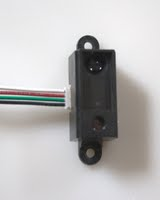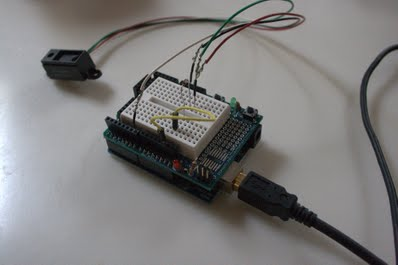## Using the GP2D02

11 Dec 2011

The GP2D02 library allows for simple communication between an arduino and one or multiple Sharp GP2D02 sensor. This library is available to download here.

The GP2D02 is a digital sensor with four connections. In this photo:• The black wire is ground.
• The green wire receives an output from the arduino.
• The red wire is 5v.
• The white wire outputs the distance to the arduino.

A diode must be included between the arduino and the sensor on the green wire.

One possible example, with pin 13 being the input from the arduino, and pin 7 being the output:To install the library, download the module, unzip it into the library folder of your arduino application.

Any code using this library must have GP2D02.h; at the beginning.

An example using this module:

This code works with the example shown above. It prints the raw range which is a number between 0 and 255.

The getRawRange() function returns a byte of the same value as that given to the arduino by the sensor. It is not formatted in any way. The closer to the sensor, the larger the number. However, the value is not linear.

Currently I am working on formatting the data to be more linear and easier to understand.

This library also contains nGP2D02 for using multiple sensors at the same time. It is very similar to the GP2D02 class, but does require slightly different syntax.

This example has six (the maximum number) sensors connected to an arduino and prints the 6 distances on the same line.

When creating a nGP2D02 object, the parameters given are two arrays of pins and an int. The int must be the length of the two arrays and equal to or less than 6.

Calling the getRawRanges method is where things begin to get a little funny. First, you must create an array of bytes of equal or greater length than the number of sensors. A pointer to the first byte in the list must then be sent to the method. The array will then be changed by the method.

nGP2D02 also has a method for collecting data from only one sensor called getRawRange(). It works in exactly the same way as getRawRange() for GP2D02, except, you must send in an int for index of the sensor that must be tested.

This example prints out the raw range for the sensor with pins (2,8)

If you have any suggestions or questions, let me know.